2015-04-23 20:13:23 chanxiaogang 阅读数 17158

1.图像频域滤波的基本概念MATLAB提供了几个和傅里叶变换相关的函数。其说明如下：
fft2(F); 二维傅立叶变换
abs(F); 获得傅立叶频谱
fftshift(F); 将变换的原点移至频率矩形的中心
ifft2(F); 二维傅立叶反变换
real(ifft2(F)); 提取变换后的实部
imag(ifft2(F)); 提取变换后的虚部
2. 频域滤波的基本步骤

（1）计算原始图像f(x,y)的DFT，得到F(u,v)。
（2）将频谱F(u,v)的零频点移动到频谱图的中心位置。
（3）计算滤波器函数H(u,v)与F(u,v)的乘积G(u,v)。
（4）将频谱G(u,v)的零频点移回到频谱图的左上角位置。
（5）计算第（4）步计算结果的傅里叶反变换g(x,y)。
（6）取g(x,y)的实部作为最终滤波后的结果图像。3 频域滤波器
1 理想低通滤波器(ILPF)D(u,v)是点(u,v)距频率原点的距离，D0为截止频率。如果图像大小为M×N，其变换亦为M×N。中心化之后，矩形中心在（M/2，N/2）2 n阶巴特沃斯低通滤波器(BLPF)n表示的是巴特沃斯滤波器的次数。随着次数的增加，振铃现象会越来越明显。与理想低通滤波器不同的是，巴特沃斯低通滤波器传递函数并不是在D0处突然不连续。对于具有平滑传递函数的滤波器，我们通常要定义一个截止频率，在该点处H(u,v)会降低为其最大值的某个给定比例。例如当D(u,v)=D0时，H(u,v)=0.5。通常，BLPF的平滑效果好于ILPF。3 高斯低通滤波器（GLPF）D0为截止频率，当D(u,v)=D0 时，滤波器下降到其最大值的0.607，无振铃现象。4 理想高通滤波器(IHPF)D(u,v)是点(u,v)距频率原点的距离，D0为截止频率。如果图像大小为M×N，其变换亦为M×N。中心化之后，矩形中心在（M/2，N/2）``````在半径为D0的圆外，所有频率没有衰减地通过滤波器，而在此半径的圆之内的所有频率完全被衰减掉。虽然这个滤波器不能使电子元件来模拟实现，但可以在计算机中用传递函数实现。由于理想高通滤波器的过度特性过于急峻，所以会产生了振铃现象。
![理想高通滤波器三维透视图](http://img.blog.csdn.net/20150423211457107)
5  n阶巴特沃斯高通滤波器(BHPF)
``````n表示的是巴特沃斯滤波器的次数。随着次数的增加，振铃现象会越来越明显。与理想高通滤波器不同的是，巴特沃斯高通滤波器传递函数并不是在D0处突然不连续。对于具有平滑传递函数的滤波器，我们通常要定义一个截止频率，在该点处H(u,v)会降低为其最大值的某个给定比例。例如当D(u,v)=D0时，H(u,v)=0.5。通常，BHPF的平滑效果好于IHPF。6 高斯高通滤波器（GHPF）``````function [U,V] = dftuv( M,N )
%DFTUV computes meshgrid frequency matrices
%set up range of variables
u=0:(M-1);
v=0:(N-1);
%compute the indices for use in meshgrid
idx=find(u > M/2);
u(idx)=u(idx)-M;
idy=find(v > N/2);
v(idy)=v(idy)-N;
%compute the meshgrid arrays
[V,U]=meshgrid(v,u);
End``````
``````function [H,D] = lpfilter( type,M,N,D0,n)
%lpfilter computes frequency domin lowpass filters
%H creates the transfer function of a lowpass filter

%use function dftuv to set up the meshgrid arrays needed for computing
%the required distances
[U,V]=dftuv(M,N);
D=sqrt(U.^2+V.^2);
%begin filter computations
switch type
case 'ideal'
H=double(D<=D0);
case 'btw'
if nargin ==4
n=1;
end
H=1./(1+(D./D0).^(2*n));
case 'gaussian'
H=exp(-(D.^2)./(2*(D0^2)));
otherwise
error('unknown filter type')
end
end``````
``````function H = hpfilter( type,M,N,D0,n )
%hpfilter computes frequency domin highpass filters
%H creates the transfer function of a highpass filter
%the transfer function Hhp of a highpass filter is 1-Hlp
%we can use function lpfilter to generate highpass filters.
if nargin==4
n=1;
end
%generate highpass filter.
hlp=lpfilter(type,M,N,D0,n);
H=1-hlp;

end``````
``````%图像频域高通滤波主程序
clear all;
F=fft2(double(I));   %快速傅里叶变换
F=fftshift(F);       %图像零频点移动到频谱图中心

[M,N]=size(F);       %计算矩阵F的大小
%调用高通滤波器函数
H=hpfilter('ideal',M,N,40);
%H=hpfilter('btw',M,N,40);
%H=hpfilter('gaussian',M,N,40);

H=fftshift(H);
G =F .* H;        %应用滤镜
%傅里叶反变换
g=ifft2(G);
%显示并比较结果
figure(1),imshow(I);
title('显示原图像');
figure(2),imshow(log(1+abs(F)),[]);
title('傅立叶变换后的频谱图');
figure(3),imshow(H,[]);
title('高通滤波器频域图像');
figure(4),imshow(log(1+abs(G)),[]);
title('高通滤波器处理后的频谱图');
figure(5),imshow(abs(real(g)),[]);
title('傅里叶反变换后的图像');``````

2019-04-27 14:59:11 GL3_24 阅读数 1324

1.如果需要看频谱图是要进行傅里叶变换的，图像的傅里叶变换其实是将图像的灰度分布函数变换为图像的频率分布函数。
2.图像的频域中的高频分量对应图像的细节信息，图像低频分量对应图像的轮廓信息。高频分量代表的是信号的突变部分（即灰度值梯度大），而低频分量决定信号的整体形象（即梯度小）。
3.在频谱图中，可以看到亮度不同的点，这些点中亮度大就证明该点的梯度大（即高频分量），亮度小证明该点的梯度小（即低频分量）。
4.频谱图中中心部分代表高频分量，四周代表低频分量，尤其是四个顶点。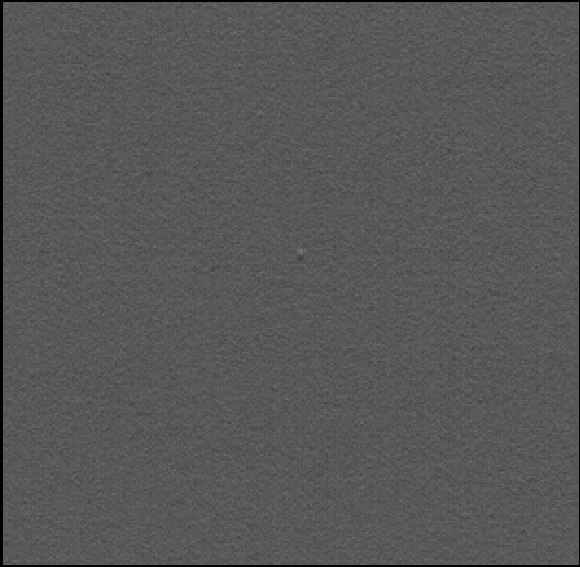``````rft_generic (Image, ImageFFT, 'to_freq', 'none', 'complex', Width)
``````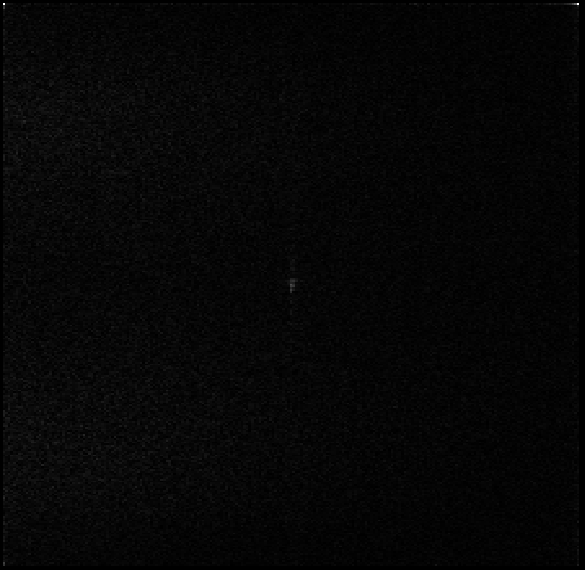``````convol_fft (ImageFFT, Filter, ImageConvol)
rft_generic (ImageConvol, ImageFiltered, 'from_freq', 'n', 'real', Width)
``````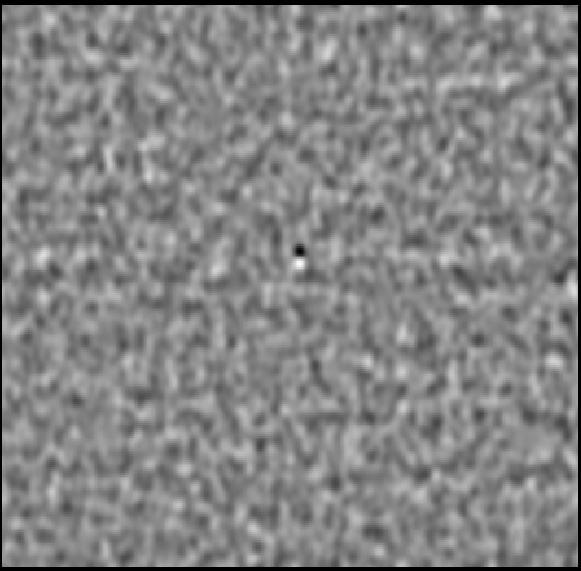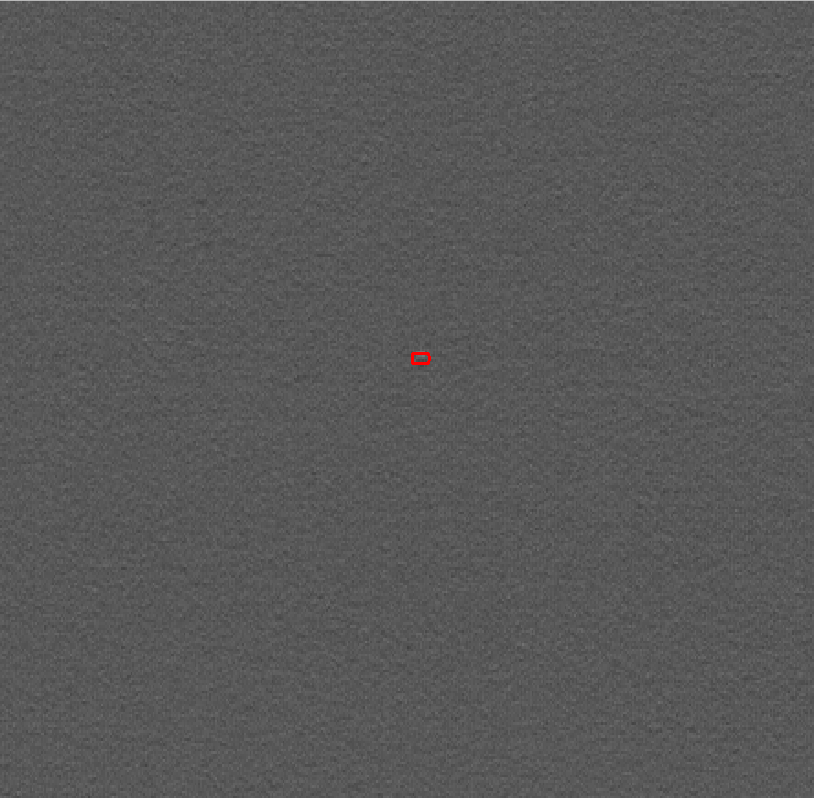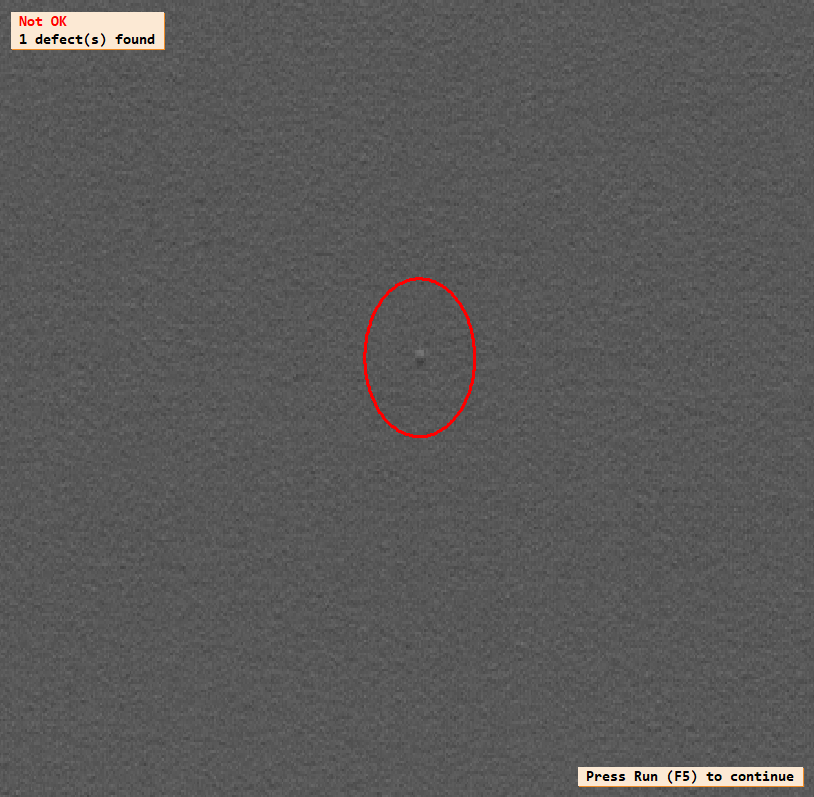2019-09-20 11:17:51 OpenSceneGraph 阅读数 225

1.频域处理基础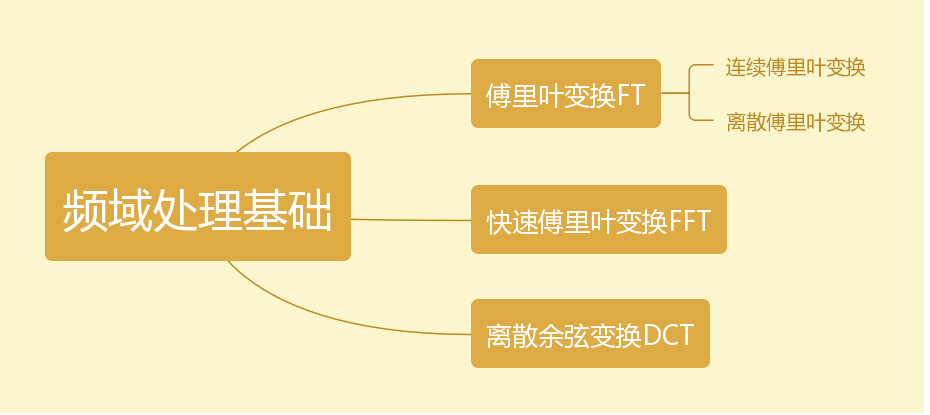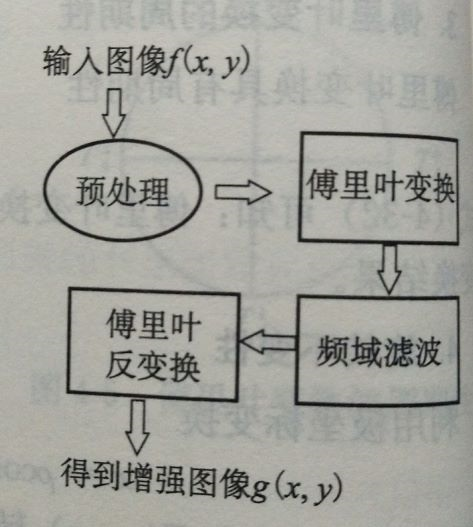上机实现：

``````m=imread('D:\Imagematlab\1.jpg');
t=rgb2gray(m);
figure;
imshow(t);
R=fftshift(fft2(t));%fft
figure;
imshow(log(abs(R)),[]);
figure;
r2=dct2(t);
imshow(log(abs(r2)),[]);%dct``````

2.空域点处理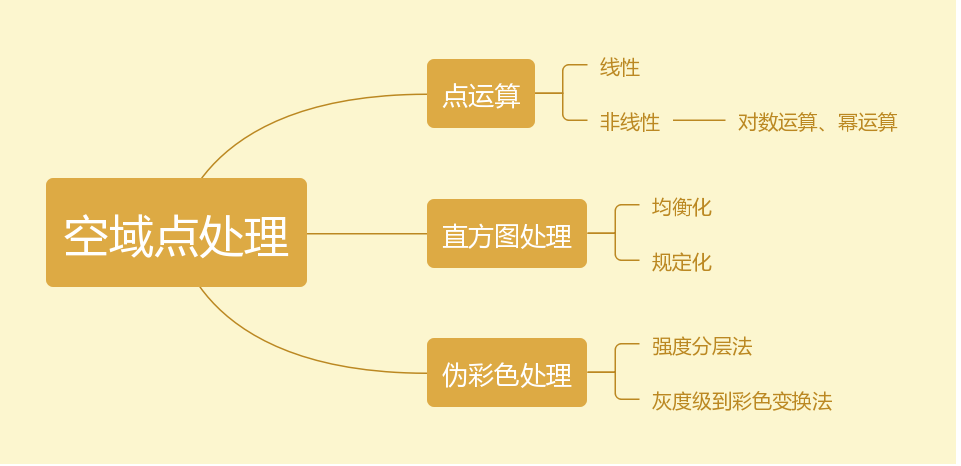伪彩色处理 or 假彩色处理：

``````I=imread('cat.jpg');
I=rgb2gray(I);
% I=im2double(I);
% a=2;b=-55;
% R=a.*I+b/255;%线性运算
% figure(1);
% imshow(R);
% R=2.5*log(I+1);%非线性运算
% figure(2);
% imshow(R);
% R=1.0*I.^4.5;%幂运算
% figure(3);
% imshow(R);

% imhist(I);
% R=histeq(I);
% figure;
% imshow(R);
% title('均衡化');

% J=imnoise(I,'salt & pepper',0.02);
% subplot(2,3,1);imshow(I);title('原图像');
% subplot(2,3,2);imshow(J);title('添加椒盐噪声');
% R1=filter2(fspecial('average',3),J);%3*3模板均值滤波
% R2=filter2(fspecial('average',5),J);
% R3=filter2(fspecial('average',7),J);
% R4=filter2(fspecial('average',9),J);
% subplot(2,3,3);imshow(uint8(R1)),title('3*3模板均值滤波');
% subplot(2,3,4);imshow(uint8(R2)),title('5*5模板均值滤波');
% subplot(2,3,5);imshow(uint8(R3)),title('7*7模板均值滤波');
% subplot(2,3,6);imshow(uint8(R4)),title('9*9模板均值滤波');
%
% figure(2);
% subplot(2,3,1);imshow(I);title('原图像');
% subplot(2,3,2);imshow(J);title('添加椒盐噪声');
% R1=medfilt2(J);
% R2=medfilt2(J,[5 5]);
% R3=medfilt2(J,[7 7]);
% R4=medfilt2(J,[9 9]);
% subplot(2,3,3);imshow(R1),title('3*3');%中值模板
% subplot(2,3,4);imshow(R2),title('5*5');
% subplot(2,3,5);imshow(R3),title('7*7');
% subplot(2,3,6);imshow(R4),title('9*9');

% figure;
% subplot(1,3,1);imshow(I);
% H=fspecial('Sobel');
% H=H';%Sobel垂直模板
% R=filter2(H,I);
% subplot(1,3,2);imshow(R,[]);
% H=H';%Sobel水平模板
% R=filter2(H,I);
% subplot(1,3,3);imshow(R,[]);
%
% figure(2);
% subplot(2,3,1);imshow(I);
% [R,t]=edge(I,'log');
% subplot(2,3,2);imshow(R);title('LOG算子检测边缘');
% R=edge(I,'Sobel');
% subplot(2,3,3);imshow(R);title('Sobel算子检测边缘');
% R=edge(I,'Prewitt');
% subplot(2,3,4);imshow(R);title('Prewitt');
% R=edge(I,'Roberts');
% subplot(2,3,5);imshow(R);title('Roberts');

``````

2019-12-11 21:32:22 weixin_44225182 阅读数 117

## 二、实验目的

1.熟悉MATLAB软件的使用。
2.掌握频域图像分析的原理及数学运算。

## 三、实验内容

1.自选一幅图像，并对其分别添加一定强度的周期噪声和高斯噪声，然后分别采用高斯模板、中值滤波的时域方法以及傅里叶变换和小波变换的频率滤波方法对该含噪图像进行去噪处理，并基于PSNR值和视觉效果这两个指标来比较这四种滤波方法对两种不同噪声的去噪能力。
2.编写一个程序，要求实现下列算法:首先将閣像分割为8x8的子图像，对每个予图像进行FFT.对每个了图像中的64个系数。按照每个系数的方差来排序后，舍去小的变換系數，只保留16个系数，实现4: I的图像压缩。
3.给定一幅行和列都为2的整数次幕图像，用Haar小波基函数对其进行二维小波变换，试着将最低尺度近似分量置零再反变换，结果是什么?如果把垂直方向的细节分量置零，反变换后结果又是什么呢?试解释一下原因。
4.基于小波变换对图像进行不同压缩比的压缩。在同压缩比情况下，对于基于小波变换和基于傅里叶变换的压缩结果，比较=二者保留原图像能里百分比情况。

Win10 64位电脑
MATLAB R2017a

## 五、实验原理

1.傅里叶变换

2.小波变换

3.PSNR算法
peak的中文意思是顶点。而ratio的意思是比率或比列的。整个意思就是到达噪音比率的顶点信号，psnr一般是用于最大值信号和背景噪音之间的一个工程项目。通常在经过影像压缩之后，输出的影像都会在某种程度与原始影像不同。为了衡量经过处理后的影像品质，我们通常会参考PSNR值来衡量某个处理程序能否令人满意。它是原图像与被处理图像之间的均方误差相对于(2n-1)2的对数值(信号最大值的平方，n是每个采样值的比特数)，它的单位是dB。

## 六、实验过程及代码

``````t=imread('a1.jpg');

%添加高斯噪声
t1=imnoise(t,'gaussian',0,0.02);
[m,n]=size(t);
t2=t;

%添加周期噪声
for i=1:m
for j=1:n
t2(i,j)=t(i,j)+30*sin(30*i)+30*sin(30*j);
end
end
imshow(t1),title('加入高斯噪声后')
figure,imshow(t2),title('加入周期噪声后');

%进行高斯滤波、中值滤波
t3_1=t;
t3_1(:,:,1)=medfilt2(t1(:,:,1),[3 3]);
t3_1(:,:,2)=medfilt2(t1(:,:,2),[3 3]);
t3_1(:,:,3)=medfilt2(t1(:,:,3),[3 3]);
figure,imshow(t3_1),title('对高斯噪声进行中值滤波','fontsize',16)
h=fspecial('gaussian',5,3);%确定滤波方式为高斯滤波 5是模板大小  3是方差
t3_2=imfilter(t2,h);%滤波操作
figure,imshow(t3_2),title('对周期噪声进行高斯滤波','fontsize',16)

t3_1=t;
t3_1(:,:,1)=medfilt2(t2(:,:,1),[3 3]);
t3_1(:,:,2)=medfilt2(t2(:,:,2),[3 3]);
t3_1(:,:,3)=medfilt2(t2(:,:,3),[3 3]);
figure,imshow(t3_1),title('对高斯噪声进行中值滤波','fontsize',16)
h=fspecial('gaussian',5,3);%确定滤波方式为高斯滤波 5是模板大小  3是方差
t3_2=imfilter(t1,h);%滤波操作
figure,imshow(t3_2),title('对周期噪声进行高斯滤波','fontsize',16)

%对周期噪声傅里叶变换滤波
t_f=rgb2gray(t2); %将图像灰度化
t_f=fft2(double(t_f));%利用fft2()函数将图像从时域空间转换到频域空间
t_f=fftshift(t_f);%将零频平移到中心位置
[m,n]=size(t_f);
m_min=round(m/2);
n_min=round(n/2);
t_rf=t_f;
d_0=100;%设置阈值
for i=1:m
for j=1:n
d_1=sqrt((i-m_min)^2+(j-n_min)^2);
if(d_1>d_0)
x=0;
else
x=1;
end
t_rf(i,j)=x*t_f(i,j);
end
end
t_rf=ifftshift(t_rf);
t_rf=uint8(real(ifft2(t_rf)));
figure,imshow(t_rf),title('对周期噪声进行傅里叶变换滤波后')

%对高斯噪声进行傅里叶变换滤波
t_f=rgb2gray(t1);
t_f=fft2(double(t_f));
t_f=fftshift(t_f);
[m,n]=size(t_f);
m_min=round(m/2);
n_min=round(n/2);
t_rf=t_f;
d_0=100;
for i=1:m
for j=1:n
d_1=sqrt((i-m_min)^2+(j-n_min)^2);
if(d_1>d_0)
x=0;
else
x=1;
end
t_rf(i,j)=x*t_f(i,j);
end
end
t_rf=ifftshift(t_rf);
t_rf=uint8(real(ifft2(t_rf)));
figure,imshow(t_rf),title('对高斯噪声进行傅里叶变换滤波后')

%对周期噪声进行小波变换滤波
[c,s]=wavedec2(t2,2,'sym4');
a1=wrcoef2('a',c,s,'sym4');
figure,imshow(uint8(a1)); title('对周期噪声进行小波变换滤波')
%对高斯噪声进行小波变换滤波
[c,s]=wavedec2(t1,2,'sym4');
a1=wrcoef2('a',c,s,'sym4');
figure, imshow(uint8(a1)); title('对高斯噪声进行小波变换滤波')
``````

SNRP算法

``````function PSNR = psnr(f1, f2)
%计算两幅图像的峰值信噪比
k = 8;
%k为图像是表示地个像素点所用的二进制位数，即位深。
fmax = 2.^k - 1;
a = fmax.^2;
MSE =(double(im2uint8(f1)) -double( im2uint8(f2))).^2;
b = mean(mean(MSE));
PSNR = 10*log10(a/b);

t=rgb2gray(t);%灰度化
[k,p]=size(t);
t=double(t)/255;%归一化 便于计算
%显示原图
imshow(t),title('原图','fontsize',16);
%利用blkproc 进行分块 并对每一块进行fft操作
t_fft=blkproc(t,[8 8],'fft2(x)');
%利用im2col进行优化操作 便于计算
t_block=im2col(t_fft,[8 8],'distinct');
[t_change,ix]=sort(t_block);%对每一块图像进行排序
[m,n]=size(t_block);
nums=48;
%对后48位系数清零
for i=1:n
t_block(ix(1:nums),i)=0;
end
t_rchange=col2im(t_block,[8 8],[k p],'distinct');
t_ifft=blkproc(t_rchange,[8 8],'ifft2(x)');%对每一块进行傅里叶反变换
figure,
imshow(t_ifft),title('4:1压缩后','fontsize',16);
``````

haar基函数进行小波变换

``````%实现一维Haar变换
function [h]=D1Haar(f,N)
J=log2(N);
Y=zeros(J,N);
f1=zeros(1,N);
H=zeros(1,N);
q=f';

%调用倒序函数
f1=Reverse(q,N);
for A=1:N
Y(1,A)=f1(A);
end

%第一层的迭代
for C=1:N/2
Y(2,C)=Y(1,C)+Y(1,C+N/2);
Y(2,C+N/2)=Y(1,C)-Y(1,C+N/2);
end

%余下层的迭代
M=0;
for B=2:J
K=2*N/(2^B);
F=zeros(1,K);
for C=1:K/2
Y(B+1,C)=Y(B,C)+Y(B,C+K/2);
Y(B+1,C+K/2)=Y(B,C)-Y(B,C+K/2);
end
for C=K+1:2*K
F(1,C-K)=Y(B,C);
end
Z=Reverse(F,K);
for D=1:K
Y(B+1,D+K)=Z(D);
end
for C=2*K+1:N
Y(B+1,C)=Y(B,C);
end
end

%系数修正
H(1,1)=(1/N)*Y(J+1,1);
H(1,2)=(1/N)*Y(J+1,2);
for a=2:J
b=2^(a-1);
c=b^(1/2);
for d=b+1:2*b
H(1,d)=(c/N)*Y(J+1,d);
end
end
h=H';

%二维哈尔函数的正变换
function [y]=D2Haar(x,M,N)
%先进行按列变换
for i=1:N
z=zeros(M,1);
for c=1:M
z(c,1)=x(c,i);
end
z1=D1Haar(z,M);%调用一维哈尔变换函数D1Haar
for c=1:M
x1(c,i)=z1(c,1);
end
end

%再进行按行变换
x2=x1';%将变换好的矩阵进行转置
for j=1:M
z=zeros(N,1);
for c=1:N
z(c,1)=x2(c,j);
end
z1=D1Haar(z,N);%调用一维哈尔变换函数D1Haar
for c=1:N
y1(c,j)=z1(c,1);
end
end
y=y1';%再最后将变换好的矩阵作转置

%对矩阵作一次哈尔变换
function [y]=D2Har(x,M,N)
for i=1:N
z=zeros(M,1);
for c=1:M
z(c,1)=x(c,i);
end
z1=D1Haar(z,M);
for c=1:M
x1(c,i)=z1(c,1);
end
end
y=x1;

%哈尔函数的逆变换
function [y]=ID2Haar(X,M,N)
har1=zeros(M);
I1=eye(M);%产生M维单位阵
har1=D2Har(I1,M,M);%调用D2Har产生M维哈尔矩阵
har2=M*har1';
I2=eye(N);%产生N维单位阵
har3=zeros(N);
har3=D2Har(I2,N,N);%调用D2Har产生M维哈尔矩阵
har4=N*har3;
y=har2*X*har4;%哈尔逆变换

%求倒叙函数
function [F]=Reverse(F,M)
N=log10(M)/log10(2);
Y=zeros(1,M);
for  x=0:M-1
A=dec2bin(x,N);%十进制转二进制
B=fliplr(A);%二进制倒叙
C=bin2dec(B);%二进制转十进制
Y(x+1)=F(C+1);
end
for x=0:M-1
F(x+1)=Y(x+1);
end

%对图片进行哈尔正变化，并对其进行恢复
clear;
M=256;
N=256;

%读取图象
subplot(2,2,1);
imshow(X);
title('原图像');

%缩放原图象
x=imresize(X,[M,N]);%将原图象缩放成分辨率为256*256的图象
subplot(2,2,2);
imshow(x);
title('缩放图象');

%对缩放图象进行Haar变换
y=D2Haar(x,M,N);
subplot(2,2,3);
imshow(y);
title('变换图象');

%对变换后的图象进行Harr逆变换，恢复原图象
z=ID2Haar(y,M,N);
%Z=imresize(z,[480,360]);
subplot(2,2,4);
imshow(z,[0,255]);
title('恢复图象');

X=rgb2gray(X);
subplot(221); imshow(X);
title('原始图像');
%对图像用小波进行层小波分解
[c,s]=wavedec2(X,2,'haar');
%提取小波分解结构中的一层的低频系数和高频系数
cal=appcoef2(c,s,'haar',1);
ch1=detcoef2('h',c,s,1);      %水平方向
cv1=detcoef2('v',c,s,1);      %垂直方向
cd1=detcoef2('d',c,s,1);      %斜线方向
%各频率成份重构
a1=wrcoef2('a',c,s,'haar',1);
h1=wrcoef2('h',c,s,'haar',1);
v1=wrcoef2('v',c,s,'haar',1);
d1=wrcoef2('d',c,s,'haar',1);
c1=[a1,h1;v1,d1];
subplot(222),imshow(c1,[]);
title ('分解后低频和高频信息');
%进行图像压缩
%保留小波分解第一层低频信息
%首先对第一层信息进行量化编码
ca1=appcoef2(c,s,'haar',1);
ca1=wcodemat(ca1,440,'mat',0);
%改变图像高度并显示
ca1=0.5*ca1;
subplot(223);imshow(cal,[]);
title('第一次压缩图像');
%保留小波分解第二层低频信息进行压缩
ca2=appcoef2(c,s,'haar',2);
%首先对第二层信息进行量化编码
ca2=wcodemat(ca2,440,'mat',0);
%改变图像高度并显示
ca2=0.25*ca2;
subplot(224);imshow(ca2,[]);
title('第二次压缩图像');
``````1.加入周期噪声、高斯噪声2.对添加了高斯噪声和周期噪声的图像进行高斯滤波PSNR值
1.对高斯噪声进行高斯滤波后 23.0287
2.对周期噪声进行高斯滤波后 23.4837

2.中值滤波PSNR值：
1.对高斯噪声进行中值滤波 23.9931
2.对周期噪声进行中值滤波 24.3134

3.傅里叶变换滤波PSNR值：
1.对添加了高斯噪声的图像进行傅里叶变换滤波 20.4922
2.对添加了周期噪声的图像进行傅里叶变换滤波 18.9736

4.小波变换滤波PSNR值：
1.对添加了高斯噪声的图像进行小波变换滤波 23.4712
2.对添加了周期噪声的图像进行小波变换滤波 24.4525

5.图像压缩(4:1压缩) 原图-左 压缩后-右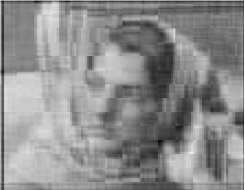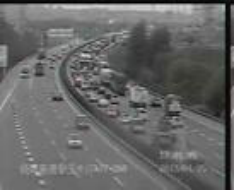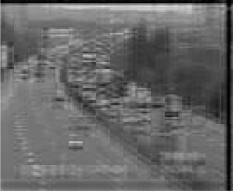1.定义

2.优点

Haar基函数进行小波变换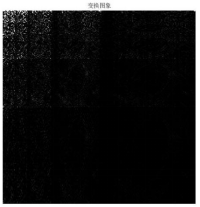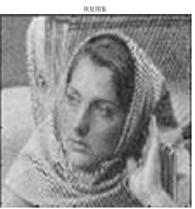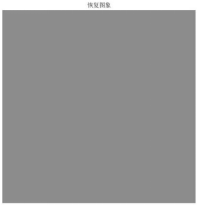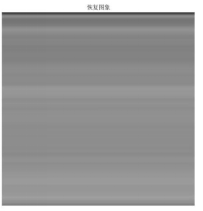1.压缩比 1:2(左-小波压缩 右-傅里叶压缩)2.压缩比 1:4(左-小波压缩 右-傅里叶压缩)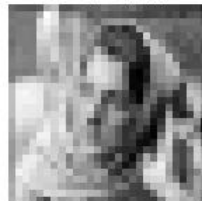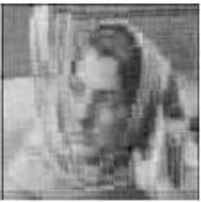## 更多

2014-07-16 15:44:59 mghhz816210 阅读数 3773

1.用（-1）的x+y次方乘以输入图像进行中心变换。

2.由（1）计算图像的DFT，即F(u,v)。

3.用滤波器函数H(u,v)乘以F(u,v)。

4.计算（3）中结果的反DFT。

5.得到（4）中结果的实部。

6.用（-1）的x+y次方乘以（5）中的结果

（以上步骤转自数字图像处理第二版（冈萨雷斯））理想低通：ILPF

巴特沃思低通：BLPF

高斯低通：GLPF

理想高通：LHPF

巴特沃思高通：BHPF

高斯高通：GHPF